# Enumeration operator

Jump to: navigation, search

A mapping of the set of all sets of natural numbers into itself (i.e. a mapping of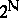into, where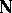is the set of natural numbers), defined as follows. Let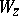be a recursively-enumerable set having Gödel number, let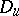be a finite set of natural numbers with canonical index(i.e.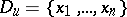, where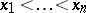and) and letbe the number of the ordered pair consisting of the numbersandfor a certain specified one-to-one recursive encoding of the pairs. Each recursively-enumerable setyields a procedure that transforms any given set of natural numbersinto the setof natural numberswith the property that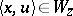for somewith, i.e.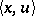belongs toand if the finite setis contained in, thenis related to. In other words,This procedure enables one to obtain effectively from any enumeration onan enumeration on. It is called an enumeration operator and is denoted by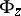. If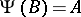for some enumeration operator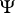, one says thatis reducible in enumerability to(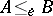).

Ifandare enumeration operators, their composite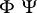is also an enumeration operator. Ifis an enumeration operator and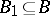, then. If, thenfor some finite set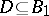. Each enumeration operatorhas a least fixed point, i.e. there exists a recursively-enumerable setsuch that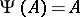, and if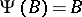, then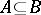.